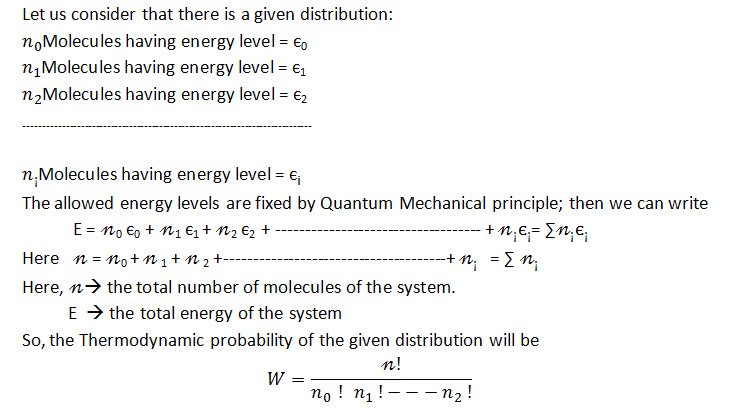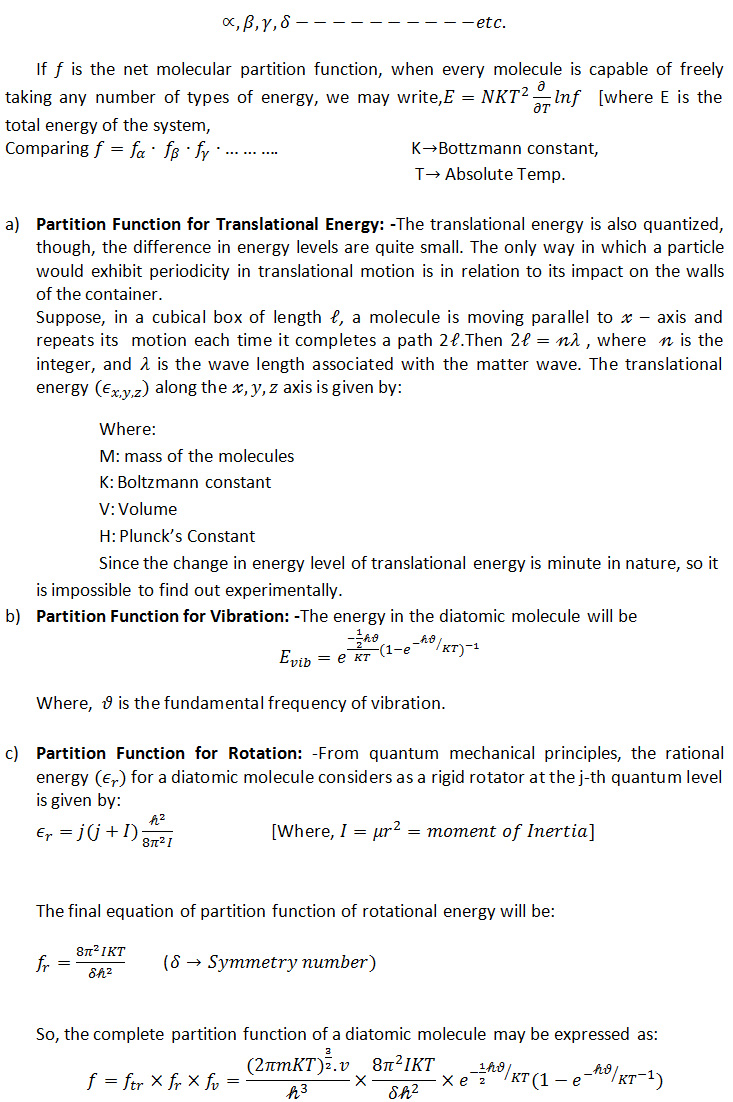APPLICATION OF THE DIFFERENT MATHEMATICAL FORMULAS OF QUANTUM MECHANICS TO EXPLAIN THIS THEORY:

1). Application of Boltzmann Distribution Law:

Succussion process is involved in drug-dynamisation; i.e. the molecules of drug and alcohol acquire random motion. Interaction between the drug and solvent molecules will be minimum due to high dynamic or kinetic energy of the drug and alcohol molecules. When the liquid becomes static or it reaches the dynamic equilibrium, the H-bonding the alcohol molecules will be dissociated and large amount of a particular energy will be released in the system due to a large number of collisions among the molecules. This energy will be taken up by the solvent molecules and a small part of it will be absorbed as an Internal energy by the alcohol molecules. Now, as the H-bonding at room temperature and pressure continues during the average life time of 1.5 Picoseconds and within such a short time the liquid rapidly reaches a state of dynamic equilibrium with Thermo-dynamically permitted minimum potential energy. So, the role of energy absorption and emission or energy transaction continues to take place in the medicinal solution at room temperature and pressure.

Since the radii of the ethyl alcohol molecules is not very large, so steric effect cannot be observed, especially in the case of comparatively higher potency, i.e. at infinite dilation. At this dilution, concentration of the drug molecules will be minimum, so the solvent behaves ideally and the interactions among the drug/drug, drug/alcohol and alcohol/alcohol will be minimum. Since, here the molecules are in random motion, so each molecule of the system attains different kinetic energy level.Now, we will have to express how energy is distributed among the vehicle molecules in the system. During drug dynamisation, the molecules of the drug and vehicle substances move here and there. So, all the molecules do not possess same energies. They possess different types of kinetic energies. i.e., their energy levels vary from each other; the total energy will be calculated by the:

‘Boltzmann Distribution Law’

Consider a system wherein the process of drug dynamisation containing n number of molecules (Drug + vehicle molecules) (n of course is very large) having total energy E takes place. Here the particles are not at the same energy level.

The distribution will be:Where the average value X ̅ of any property X of a system governed by position and momenta co-ordinates is obtained by the above equation where n be the total number of molecules.

So,Where ε is the energy

K is the Boltzmann Constant

T is the absolute temperature,

dq1, dps etc. are the momentum Co-ordinates around the space.

So, from the above equation, we can evaluate the distribution of the energy among the vehicle molecules, average velocity, momenta etc. of drug and vehicle molecules during drug dynamisation.

2) Application of Schrödinger’s wave equation: Since the theory is fully associated with quantum mechanics, wave mechanics and statistical mechanics, so the Schrödinger’s wave equation is applicable here to calculate the amount of different types of energies (vibrational energy, rotational energy, translational energy, energy of resonance, electromagnetic energy etc.

3) Application of partition functions: During drug dynamisation (succession), there occurs molecular collisions amongst drug vs drug, alcohol vs alcohol, alcohol vs drug, alcohol vs walls of the container, drug vs container due to random motions of drug and vehicle molecules, so different types of energies are produced. Fraction of molecules which is imparted to involve the energy changes is called partition functions.

Let us consider the total number of molecules are N, Volume of the container is V. In expressing the partition function, all the types of energy have to be considered. Vibrational, Rotational, Translational, Electromagnetic, Resonating etc. energy changes take place.

Let the different types of energy be labeled as:Partition functions are used to calculate the fractional amount of different types of energy imparted by the drug molecules to undergo the Vibrational, Rotational, Translational energy changes in the system.

DIFFERENT TYPES OF RELATION OF PARTITION FUNCTION WITH DIFFERENT PARAMETERS

To be continued…..
Share.